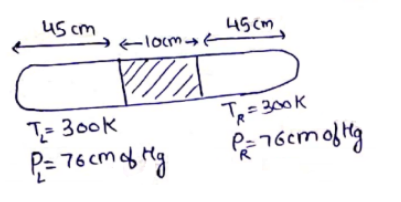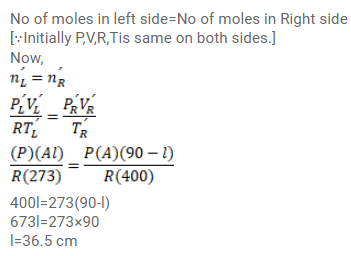# Use R=8.3 J/mol-K wherever required. A glass tube, sealed at both ends, is 100 cm long.Question:

Use $\mathrm{R}=8.3 \mathrm{~J} / \mathrm{mol}-\mathrm{K}$ wherever required.

A glass tube, sealed at both ends, is $100 \mathrm{~cm}$ long. It lies horizontally with the middle $10 \mathrm{~cm}$ containing mercury. The two ends of the tube contain air at $27^{\circ} \mathrm{C}$ and at a pressure $76 \mathrm{~cm}$ of mercury. The air column on one side is maintained at $0^{\circ} \mathrm{C}$ and the other side is maintained at $127^{\circ} \mathrm{C} .$ Calculated the length of the air column on the cooler side. Neglect the changes in the volume of mercury and of the glass.

Solution: